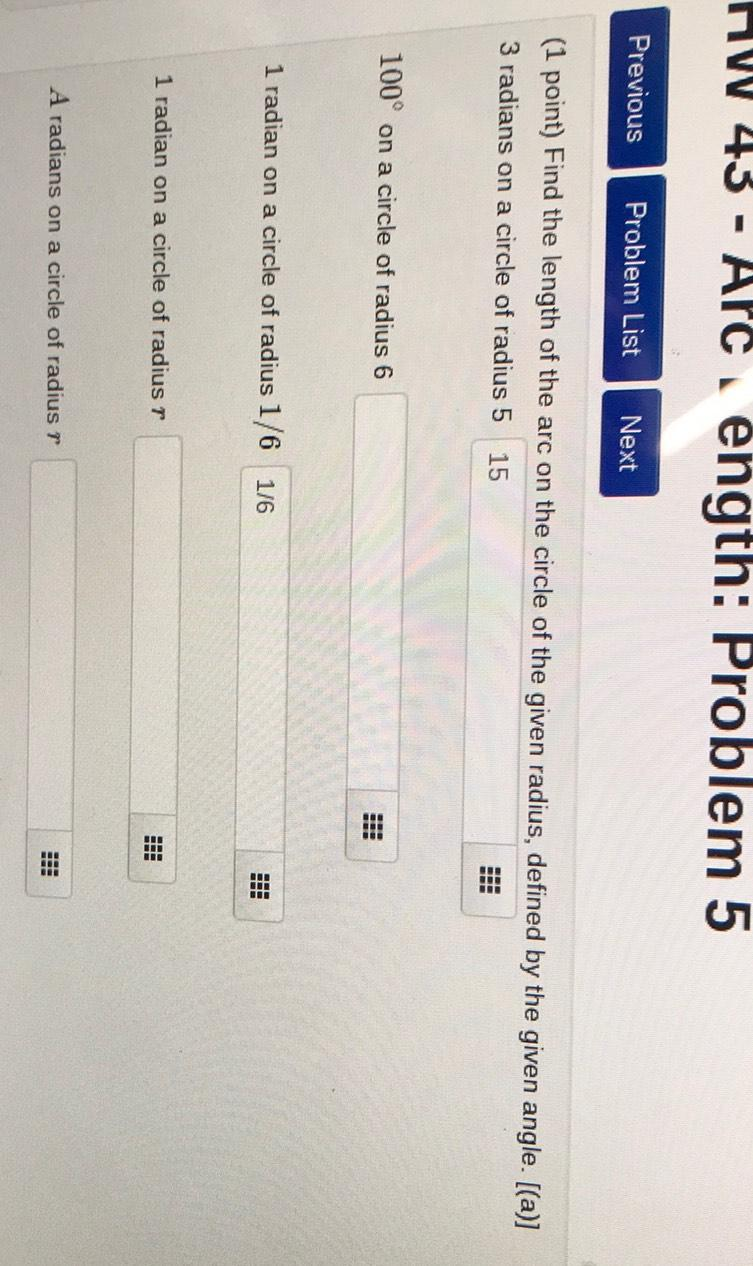Question:

HW 43 - APC ength: Problem 5 Previous Problem List Next (1 point) Find the length of the arc on the circle of the given radius,HW 43 - APC ength: Problem 5 Previous Problem List Next (1 point) Find the length of the arc on the circle of the given radius, defined by the given angle. [(a)] 3 radians on a circle of radius 5 15 OD 100° on a circle of radius 6 ID 1 radian on a circle of radius 1/6 1/6 B 1 radian on a circle of radius r A radians on a circle of radius r